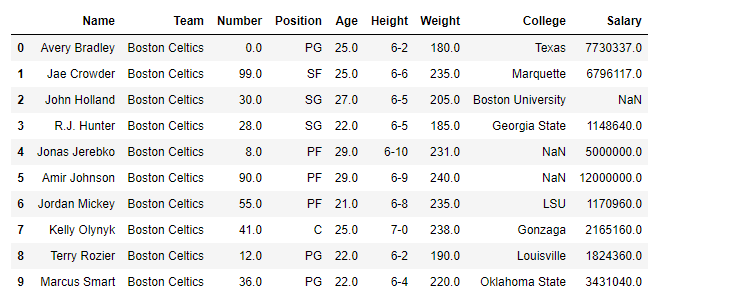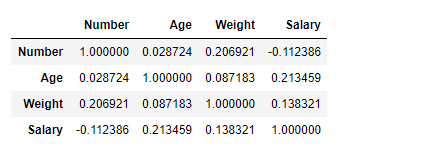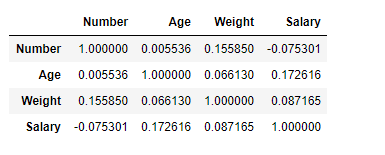# Python | Pandas dataframe.corr()

Python is a great language for doing data analysis, primarily because of the fantastic ecosystem of data-centric python packages. Pandas is one of those packages and makes importing and analyzing data much easier.

Pandas` dataframe.corr()` is used to find the pairwise correlation of all columns in the dataframe. Any `na` values are automatically excluded. For any non-numeric data type columns in the dataframe it is ignored.

Syntax: DataFrame.corr(self, method=’pearson’, min_periods=1)

Parameters:
method :
`pearson `: standard correlation coefficient
`kendall `: Kendall Tau correlation coefficient
`spearman `: Spearman rank correlation
min_periods : Minimum number of observations required per pair of columns to have a valid result. Currently only available for pearson and spearman correlation

Returns: count :y : DataFrame

Note: The correlation of a variable with itself is 1.

Example #1: Use `corr()` function to find the correlation among the columns in the dataframe using ‘Pearson’ method.

 `# importing pandas as pd ` `import` `pandas as pd ` ` `  `# Making data frame from the csv file ` `df ``=` `pd.read_csv(``"nba.csv"``) ` ` `  `# Printing the first 10 rows of the data frame for visualization ` `df[:``10``] `Now use `corr()` function to find the correlation among the columns. We are only having four numeric columns in the dataframe.

 `# To find the correlation among ` `# the columns using pearson method ` `df.corr(method ``=``'pearson'``) `

Output :The output dataframe can be interpreted as for any cell, row variable correlation with the column variable is the value of the cell. As mentioned earlier, that the correlation of a variable with itself is 1. For that reason all the diagonal values are 1.00

Example #2: Use `corr()` function to find the correlation among the columns in the dataframe using ‘kendall’ method.

 `# importing pandas as pd ` `import` `pandas as pd ` ` `  `# Making data frame from the csv file ` `df ``=` `pd.read_csv(``"nba.csv"``) ` ` `  `# To find the correlation among ` `# the columns using kendall method ` `df.corr(method ``=``'kendall'``) `

Output :The output dataframe can be interpreted as for any cell, row variable correlation with the column variable is the value of the cell. As mentioned earlier, that the correlation of a variable with itself is 1. For that reason all the diagonal values are 1.00.

My Personal Notes arrow_drop_upCheck out this Author's contributed articles.

If you like GeeksforGeeks and would like to contribute, you can also write an article using contribute.geeksforgeeks.org or mail your article to contribute@geeksforgeeks.org. See your article appearing on the GeeksforGeeks main page and help other Geeks.

Please Improve this article if you find anything incorrect by clicking on the "Improve Article" button below.

Improved By : pratibha_gupta

Article Tags :

3

Please write to us at contribute@geeksforgeeks.org to report any issue with the above content.